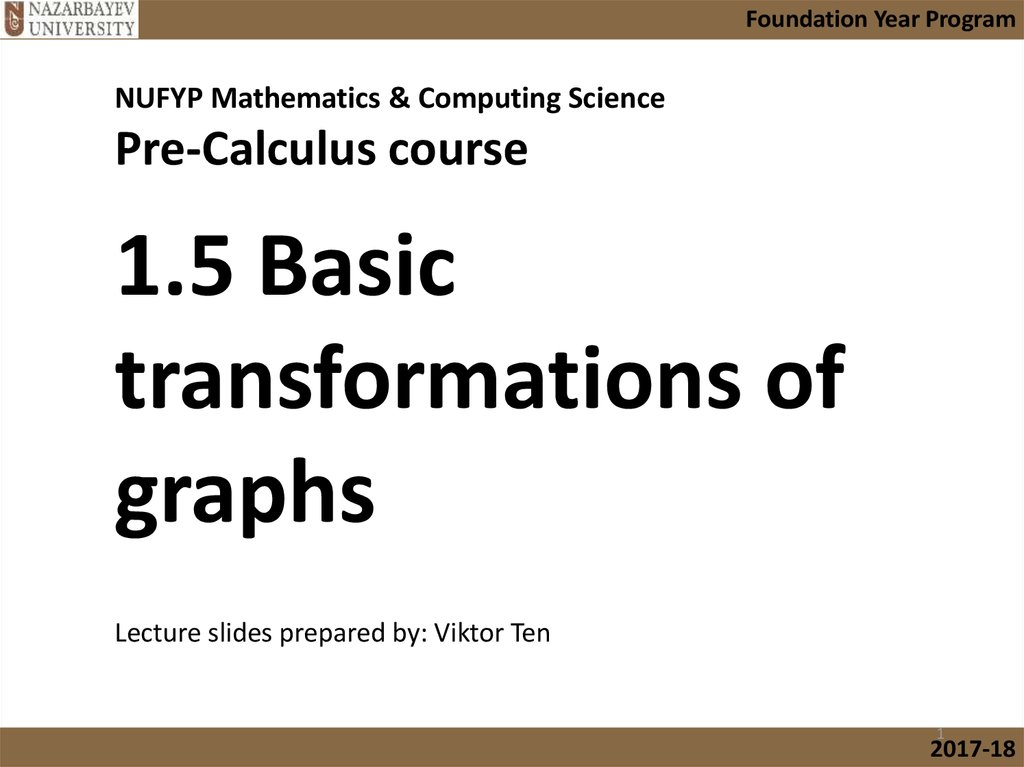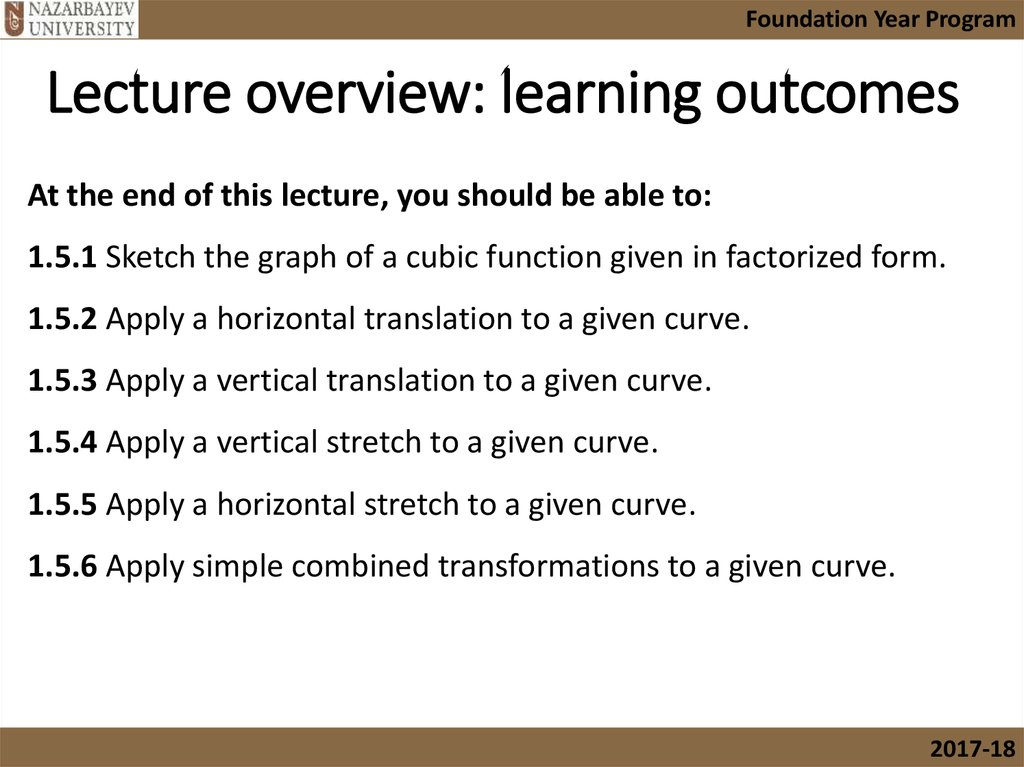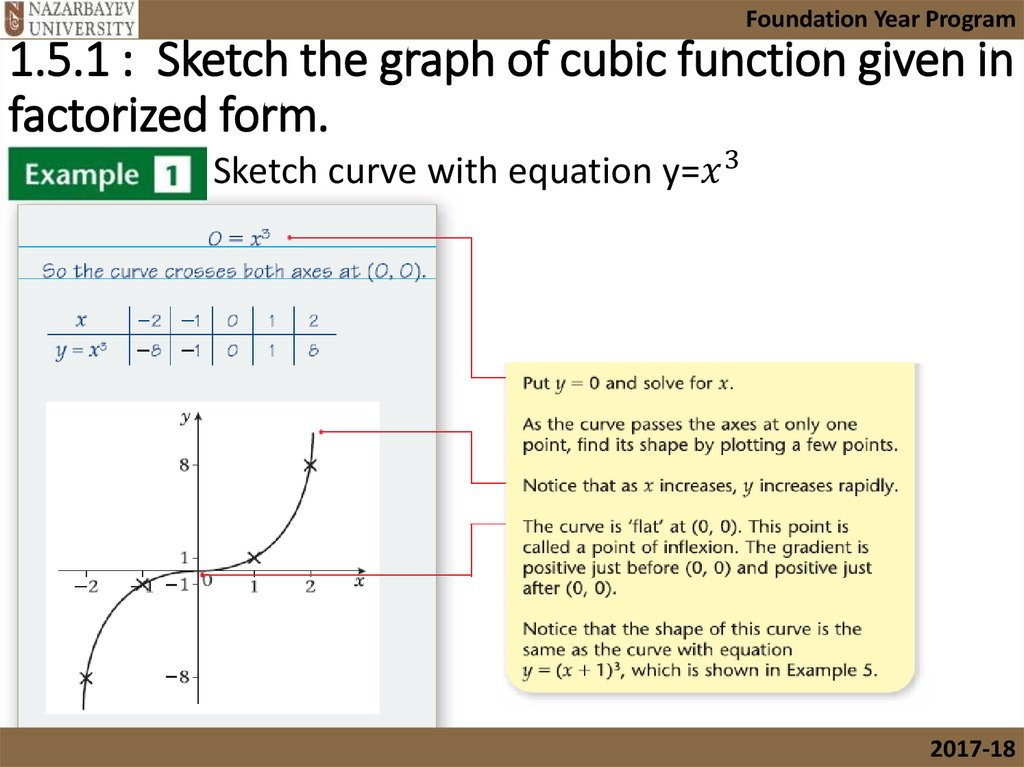# Basic transformations of graphs

## 1.

Foundation Year Program
NUFYP Mathematics & Computing Science
Pre-Calculus course
1.5 Basic
transformations of
graphs
Lecture slides prepared by: Viktor Ten
1
2017-18

## 2. Lecture overview: learning outcomes

Foundation Year Program
Lecture overview: learning outcomes
At the end of this lecture, you should be able to:
1.5.1 Sketch the graph of a cubic function given in factorized form.
1.5.2 Apply a horizontal translation to a given curve.
1.5.3 Apply a vertical translation to a given curve.
1.5.4 Apply a vertical stretch to a given curve.
1.5.5 Apply a horizontal stretch to a given curve.
1.5.6 Apply simple combined transformations to a given curve.
2017-18

## 3. 1.5.1 : Sketch the graph of cubic function given in factorized form.

Foundation Year Program
1.5.1 : Sketch the graph of cubic function given in
factorized form.
Sketch curve with equation y=